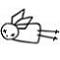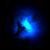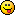Discussion about math, puzzles, games and fun.   Useful symbols: ÷ × ½ √ ∞ ≠ ≤ ≥ ≈ ⇒ ± ∈ Δ θ ∴ ∑ ∫  π  -¹ ² ³ °

You are not logged in.

#1 2006-03-29 10:02:59

MathsIsFunRegistered: 2005-01-21
Posts: 7,691

Plane Geometry Formulas

Plane Geometry Formulas

"The physicists defer only to mathematicians, and the mathematicians defer only to God ..."  - Leon M. Lederman

Offline

#2 2006-04-02 01:37:15

ganeshRegistered: 2005-06-28
Posts: 28,275

Re: Plane Geometry Formulas

Triangle:-
The area of a triangle, A is given by the formula

where b is the base and h is the height or the altitude.
If the lengths of the three sides of a triangle are known,  the area can be calculated using Hero's formula.

where a,b,c are the three sides of the triangle and s is the semi-perimeter,

If the three sides of the triangle are equal, the triangle is called an equilateral triangle and each angle is 60 degrees.
The area of an equilateral triangle is given by the formula

where a is the length of each side.
The altitude or height, h, of an equilateral triangle is

and the perimeter is
.

Square:-
A square consists of four equal sides and each side is perpendicular to the other, and opposite sides are parallel.
If a is the length of the side of a square, the are A is

Perimeter = 4a
Length of the diagonal is equal to

Rectangle.
A rectangle is a quadrilateral with opposite sides parallel to each other and each side perpendicular to the adjacent side. The opposite sides are equal.

Area = l x b (where l and b are the sides)
Perimeter = 2(l+b)
Length of the diagonal =

It is no good to try to stop knowledge from going forward. Ignorance is never better than knowledge - Enrico Fermi.

Nothing is better than reading and gaining more and more knowledge - Stephen William Hawking.

Offline

#3 2006-04-03 03:28:42

ganeshRegistered: 2005-06-28
Posts: 28,275

Re: Plane Geometry Formulas

Circle
Area of a circle, A

Circumference of a circle =

Area of a semicircle =

It is no good to try to stop knowledge from going forward. Ignorance is never better than knowledge - Enrico Fermi.

Nothing is better than reading and gaining more and more knowledge - Stephen William Hawking.

Offline

#4 2006-04-03 03:40:59

Patrick
Real MemberRegistered: 2006-02-24
Posts: 1,005

Re: Plane Geometry Formulas

Parallelogram
Area of a trapezoid, A

There is also a formula for the area of a trapezoid, where the length of all sides are known:

http://en.wikipedia.org/wiki/Trapezoid wrote:

Another formula for the area can be used when all that is known are the lengths of the four sides. If the sides are a, b, c and d, and a and c are parallel (where a is the longer parallel side), then:

Last edited by Patrick (2006-04-03 03:42:02)

Support MathsIsFun.com by clicking on the banners.
What music do I listen to? Clicky click

Offline

#5 2006-04-07 18:01:16

ganeshRegistered: 2005-06-28
Posts: 28,275

Re: Plane Geometry Formulas

Regular Polygons

A polygon is formed by a closed series of straight lines (segments).
A regular polygon is one with all sides and angles equal.
An equilateral triangle, a square etc. are examples of regular polygons.

The Sum of all angles of a polygon with 'n' sides is

in degrees.

Sum of all the exterior angles = 360°

No. of sides = 360°/Exterior angle.

where n=no. of sides and s=length of each side.

Perimeter = n x s

No. of diagonals =

It is no good to try to stop knowledge from going forward. Ignorance is never better than knowledge - Enrico Fermi.

Nothing is better than reading and gaining more and more knowledge - Stephen William Hawking.

Offline

#6 2006-04-07 18:46:18

ganeshRegistered: 2005-06-28
Posts: 28,275

Re: Plane Geometry Formulas

Area = 1/2 x (Product of diagonals) x (sine of the angle between them)

Area = 1/2 x diagonal x sum of the perpendiculars to it from opposite sides.

Parallelogram

Area = Base x Height

Area = Product of any two adjacent sides x (sine of the included angle)

Perimter = 2 (a+b) where a and b are the adjacent sides.

Rhombus

Area = 1/2 x Product of the diagonals

Area = Product of the adjacent sides x sine of the angle between them.

Trapezium

Area = 1/2 x (Sum of the parallel sides) x (height)

Regular Hexagon

where a=length of a side.

It is no good to try to stop knowledge from going forward. Ignorance is never better than knowledge - Enrico Fermi.

Nothing is better than reading and gaining more and more knowledge - Stephen William Hawking.

Offline

#7 2006-04-07 22:42:24

ganeshRegistered: 2005-06-28
Posts: 28,275

Re: Plane Geometry Formulas

Circle (cont'd)

Length of an Arc

Sector of a circle is the area of a circle between two radii.
Area of a sector of a circle.

where l is the length of the arc.

Segment:- A sector minus the triangle formed by the two radii is called the segment. Area of the segment,

Perimeter of the segment :- Length of the arc + length of the chord

It is no good to try to stop knowledge from going forward. Ignorance is never better than knowledge - Enrico Fermi.

Nothing is better than reading and gaining more and more knowledge - Stephen William Hawking.

Offline

#8 2006-08-05 13:44:23

Zhylliolom
Real MemberRegistered: 2005-09-05
Posts: 412

Re: Plane Geometry Formulas

Circle Inscribed in a Triangle

The radius of a circle inscribed in a triangle of sides a, b, and c is given by

where the semiperimeter s of the triangle is given by

Circle Circumscribing a Triangle

The radius of a circle circumscribing a triangle of sides a, b, and c is given by

where once again s is the semiperimeter of the triangle.

Regular n-gon Inscribed in a Circle

The area of a regular n-gon inscribed in a circle of radius r is given by

The perimeter of the n-gon is given by

Regular n-gon Circumscribing a Circle

The area of a regular n-gon circumscribing a circle of radius r is given by

The perimeter of the n-gon is given by

Ellipse

The area of an ellipse of semi-major axis a and semi-minor axis b is given by

The perimeter of the ellipse is given by

or approximately

Offline

#9 2007-08-11 22:40:27

Identity
MemberRegistered: 2007-04-18
Posts: 934

Re: Plane Geometry Formulas

Area of a regular n-gon if the side length is known

Where n is the number of sides of the n-gon, and s is the side length.

Area of a regular n-gon if the diagonal length is known (formulated myself):

Where n is the number of sides of the n-gon and R is the length of a diagonal.

(Oh I see ganesh has the first formula in a different form)

Last edited by Identity (2007-08-12 04:51:21)

Offline

#10 2009-01-13 23:50:15

ganeshRegistered: 2005-06-28
Posts: 28,275

Re: Plane Geometry Formulas

Rhombus:

Area, A is given by

where
are the two diagonals.

The perimeter, P, is given by the formula

It is no good to try to stop knowledge from going forward. Ignorance is never better than knowledge - Enrico Fermi.

Nothing is better than reading and gaining more and more knowledge - Stephen William Hawking.

Offline

#11 2009-01-14 00:01:37

ganeshRegistered: 2005-06-28
Posts: 28,275

Re: Plane Geometry Formulas

Ring:
The area, A, of a ring is given by the formula

where R is the radius of the outer circle and r is the radius of the inner circle.

It is no good to try to stop knowledge from going forward. Ignorance is never better than knowledge - Enrico Fermi.

Nothing is better than reading and gaining more and more knowledge - Stephen William Hawking.

Offline

#12 2009-01-14 03:49:03

JaneFairfax
MemberRegistered: 2007-02-23
Posts: 6,868

Re: Plane Geometry Formulas

ganesh wrote:

Length of the side, s is given by

This is wrong! It is

Offline

#13 2009-01-14 03:53:05

JaneFairfax
MemberRegistered: 2007-02-23
Posts: 6,868

Re: Plane Geometry Formulas

More Rhombus properties:

Let
be a non-obtuse interior angle of a rhombus with side
and diagonals
and
.

Then

(i) If you fix

and vary
, the sum of the squares of the diagonals is constant. Indeed
.

(ii) If you fix

and vary
, the ratio of the diagonals is constant. Indeed
.

Offline

#14 2009-01-14 18:29:12

ganeshRegistered: 2005-06-28
Posts: 28,275

Re: Plane Geometry Formulas

JaneFairfax,
Thanks for post #12. I have edited post #10.

It is no good to try to stop knowledge from going forward. Ignorance is never better than knowledge - Enrico Fermi.

Nothing is better than reading and gaining more and more knowledge - Stephen William Hawking.

Offline

#15 2009-03-12 18:16:05

ganeshRegistered: 2005-06-28
Posts: 28,275

Re: Plane Geometry Formulas

Circumscribed Circle
The circle which passes through the vertices of a triangle is called the circumscribing circle or circumcircle. The center of the circle is called the circumcenter and the radius of the circle is called the circumradius of the triangle.

If R is the circumradius of the triangle,

where Δ is the area of the triangle.

Inscribed Circle
A circle which touches the three sides of a triangle a, b, and c internally is called an inscribed circle or incircle. The centerof this circleis called the incenter and the radius of the circle is called the inradius.
If r be the radius, then

.

.

Radii of Inscribed and Circumscribed Circles
The radius of the inscribed circle r of a regular polygon is given by

The Radius of a circumscribed circle of a regular polygon is given by

.

Area of a Regular Polygon

It is no good to try to stop knowledge from going forward. Ignorance is never better than knowledge - Enrico Fermi.

Nothing is better than reading and gaining more and more knowledge - Stephen William Hawking.

Offline